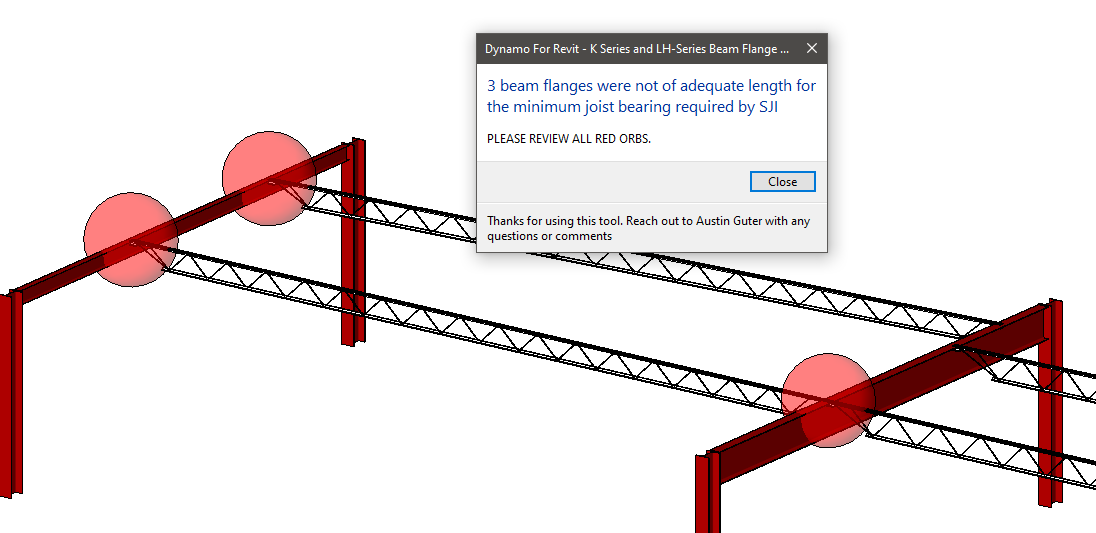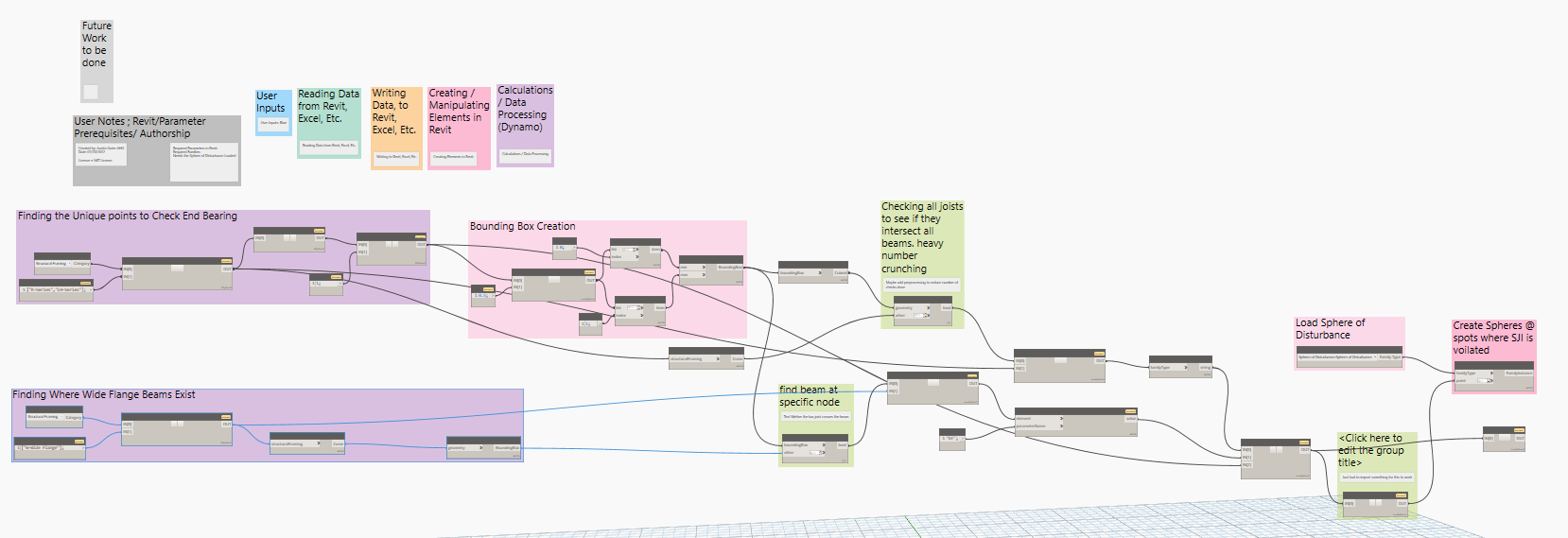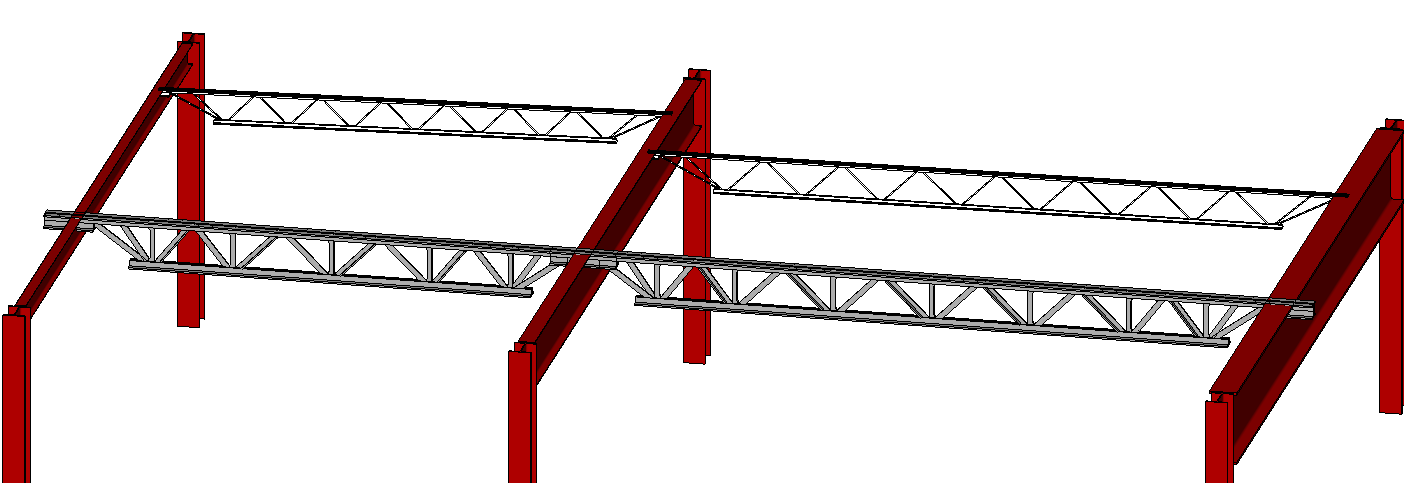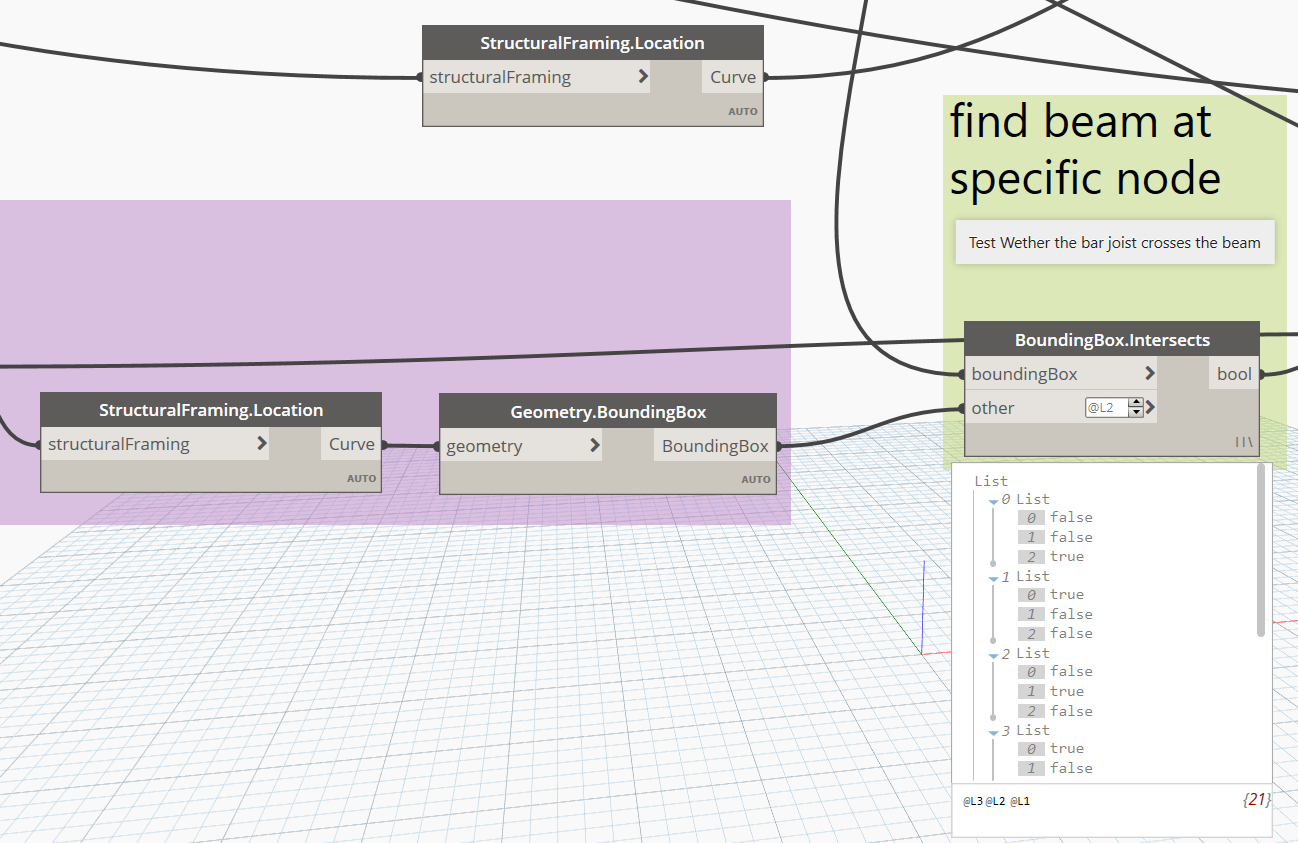A co-worker of mine asked if I could find a way to check min. joist bearing lengths after being burned on a recent project where they had a few beams that worked for strength and deflection, but did not match SJI's minimum flange dimensions for bearing.Tedious, easy problems like this is where dynamo really shines, lets dig into the code to make this happen.

##### Step 1 - Collect All Bar Joists

The dynamo graph located on my github works by pulling all the K-Series Joists and LH-Series Joists in the current 3d view.If you have never seen a dynamo graph before, above is an image of the code used to make joist bearing length checker. I believe the term is visual programming, where you connect code bits with wires and it creates a nice visual programming look.

Please note when using the tool, it will only work based on the elements in your current view (I usually run this dynamo graph in a 3d view).

This bit of code gathers all of the K  and LH Series Joists in the project.

```import clr
from Autodesk.Revit.DB import *
from RevitServices.Persistence import DocumentManager

doc = DocumentManager.Instance.CurrentDBDocument

category = UnwrapElement(IN)
joists = IN
elements = FilteredElementCollector(doc, doc.ActiveView.Id)\
.OfCategoryId(category.Id)\
.WhereElementIsNotElementType()

filtered = []
for e in elements:
type_id = e.GetTypeId()
element_type = doc.GetElement(type_id)
family = element_type.Family
name = family.Name
if joists in name or joists in name:
filtered.append(e)

OUT = filtered
```
##### Step 2 - Check for Locations where Joist Bearing Should be checked.

Above identifies the locations where the program might want to check joist bearing, but we want to reduce the number of points the program will check if we have (2) joists bearing on the same girder.

The image below will be used in the post, note that there are  (4) total joists in the sample, the top two joists being K Series Joists, with an offset in plane, and the bottom two joists being LH Series joist, parallel to each other.The first part of the code will identify (2) unique points for each end of each bar joist, giving a total of (8) total points. In this example though, I want to filter out the location where both LH-series joists are parallel, as this will be a location where the program should spit out only (1) unique point due the joist occurring at the same locatoin, requiring double the minimum bearing length:

This bit of code filters out points if they are close to each other:

```pnts = IN
tol = IN
# Place your code below this line
def distance(point1,point2) :
x1 = point1.X
y1 = point1.Y
z1 = point1.Z
x2 = point2.X
y2 = point2.Y
z2 = point2.Z
dist = ((x2-x1)**2+(y2-y1)**2+(z2-z1)**2)**0.5
return dist
uni_pnts = []
temp_pnts = pnts
while len(temp_pnts) >= 1:
if len(temp_pnts) != 1:
pnt_init = temp_pnts
rem_pnts = temp_pnts[1:]
j = 0
on_off = 1
while on_off >= 0.5:
delta = distance(pnt_init,rem_pnts[j])
if delta <= tol :
uni_pnts.append(pnt_init)
temp_pnts.pop(j+1)
temp_pnts.pop(0)
on_off = 0
elif j == len(rem_pnts)-1:
uni_pnts.append(pnt_init)
temp_pnts.pop(0)
on_off = 0
else:
j+=1
else:
uni_pnts.append(temp_pnts)
temp_pnts.pop(0)
# Assign your output to the OUT variable.
OUT = uni_pnts
```

Given that the program will run for an unknown amount times, we need to utilize a while loop and remove items from our list as they go, python's .pop() function is quite useful to do this.

In our sample above, it takes the number of points from (8) to (7) as it recongnizes the (2) LH series joists being co-planar. You can see in my poor sketch below, the program effectively realizes the red points of 6 and 7 are close enough and conisders them to be a double bar joist location now.##### Step 3 - Make A bounding box at each of the purple nodes

A bounding box in dynamo is quite useful for checking if geometries intersect with other geometries, in our case, we need to check if the bounding box at the purple nodes crosses any beams. Bounding boxes in dynamo show up in this orange color by default. See belowIn order to do this, we use dynamo list lacing and change it to longest to check all purple bounding boxes and see if they cross any of the wide flange framing. If they do, we need to check our SJI minimum bearing lengths verse the wide flange width. In the snip below, each of the (7) purples nodes identified above are checked to see if they cross any wide flange beam. In our test model, there are only (3) beams, so 7*3 = 21 true-false results are returned. Where a true occurs, a joist is supported by the corresponding wide flange beam.This is one area that I would like to revisit, in larger models, I am sure this task that gets computationally expensive, and quickly. If anyone has any ideas on a cool algorithm to tackle this part of the program, please let me know. I plan to explore this further in the future.

##### Part 4 - Testing Beam Widths vs. SJI Minimums

Now that we have idenified where double and single joist connections occur, in addition to the beam that supports the specific joist, we can now check SJI Bearing lengths, 4" minimum for a K Series joist and 6" minimum for an LH Series joist.

This is done with a long if statement shown below:

```# The inputs to this node will be stored as a list in the IN variables.
joist_types = IN
beam_widths = IN
pnts = IN

pnts_failing = []
for ind, (joist,beam_width) in enumerate(zip(joist_types,beam_widths)):
if len(joist)==1:
if "K" in joist: #SJI Min Bearing for K Series is 4"
min_seat = 0.3333
if beam_width <= min_seat:
pnts_failing.append(pnts[ind])
else:
pass
else:
min_seat = 0.5 #SJI Min Bearing for LH Series is 6"
if beam_width <= min_seat:
pnts_failing.append(pnts[ind])
else:
pass
else:
if "LH" in joist or "LH" in joist: #SJI Min Bearing for (2) LH Series is 12"
min_seat = 1
if beam_width <= min_seat:
pnts_failing.append(pnts[ind])
else:
pass
else:
min_seat = 0.67 #SJI Min Bearing for (2) K Series is 8"
if beam_width <= min_seat:
pnts_failing.append(pnts[ind])
else:
pass

```
##### Part 5 - Visualize the Results

The program places red orbs at locations where minimum joist bearing is not met. In our example the central girder is a W18x35, bf = 6". A singular red orb is placed at the LH-Series location as the LH location would need 12" of flange width to meet SJI requirements.A nice pop up window stating how many joists did not have adequate joist bearing is also displayed.

Hopefully this example demonstrates a useful way to utilize dynamo. Let me know how you use dynamo, it is a great way to automate boring, time consuming tasks that need be completed.